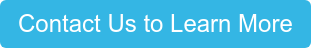## What is debt yield?

Debt yield is the return that a lender would receive if the borrower defaulted on the loan and the lender had to foreclose on the subject property. This is a simple metric used to determine the risk of a proposed loan. It’s also used to ensure a loan amount isn’t inflated by low market cap rates, low interest rates, or high amortization periods, which can all skew other analysis metrics such as Loan-to-Value.

## Debt yield calculation

The formula for debt yield is:

Debt Yield = Net Operating Income (NOI) / Loan Amount

While it’s very easy to calculate, the lender must determine if the result is a worthwhile investment for them, given the property type and market conditions. Essentially, the lower the Debt Yield the higher the lender’s risk. Generally, ten percent (10%) is considered the minimum Debt Yield for a loan.

Debt Yield is calculated independently of capitalization rates (cap rate), interest rates, or amortization periods. It’s a quick and easy way to assess the risk of a loan, but shouldn’t be the only criteria in analyzing one.

### Other loan analysis criteria

Two other metrics for evaluating a loan are Loan-to-Value (LTV) and Debt Service Coverage Ratio (DSCR). A proposed loan must be supported by Debt Yield and comply with LTV and DSCR requirements as determined by the lender.

For example, DSCR can be adjusted to fit a lender’s “box” by changing the amortization period.  In the table below, expanding the amortization period by five (5) years from 20 years to 25 years increases the DSCR from 1.15x to 1.22x. If the lender had a 1.20x DSCR requirement, that one small adjustment can make the difference in getting a loan done or not.

 Loan Amount \$750,000 \$750,000 Interest Rate 10.0% 10.0% Amortization 20 25 Loan PMT \$86,852 \$81,783 DSCR 1.15x 1.22x

The adjustment to the amortization period does not impact the Debt Yield. In the example (assumes NOI of \$100,000, a 10% cap rate, and 75% LTV) above, the Debt Yield would be calculated as follows:

Debt Yield = Net Operating Income (NOI) / Loan Amount

Debt Yield= \$100,000/\$750,000

Debt Yield= 13.33%

## Why lenders use debt yield

Debt Yield provides a lender a measure of risk independent of interest rate and amortization periods, which DSCR and LTV don’t allow for. Given the recent market conditions (pre-COVID-19), where low interest rates and compressed cap rates were the norm, loan amounts determined solely by DSCR and LTV provided little comfort in a rising interest rate environment.Topics: Small Balance Commercial